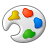Announcements
The way to achieve your own success is the willingness to help somebody else. Go for it!
cancel
Showing results for
Did you mean:Contributor III

## Help with nested aggregation

Hello!  I'm trying to figure out how to modify this expression so that qliksense accepts the formula (as nested aggregation is not allowed).  Basically, if an average of scores is greater than or equal to 3, I want that to generate a count of "1", and then be able to sum those 1s (so that this will translate to a pivot format).  Here's my formula:

Sum(IF(Avg({\$<[ELPScores.Concept]={'1.1*','1.2*','2.1*','2.2*','2.4*','3.1*','4.2*'}>} IndicatorScores)>=3.0,1))

Thanks for the help!

Katie

2 RepliesContributor III
Author

Wait- I think I may have got it after reviewing some other articles...!

SUM(IF(aggr(Avg({\$<[ELPScores.Concept]={'1.1*','1.2*','2.1*','2.2*','2.4*','3.1*','4.2*'}>} IndicatorScores)>=3.0,Key_UserID),1))

Thanks Qlik community!MVP

You need to Add Aggr() between your two aggregating functions (Sum and Avg) with all your pivot table dimensions.

Sum(Aggr(

If(Avg({\$<[ELPScores.Concept]={'1.1*','1.2*','2.1*','2.2*','2.4*','3.1*','4.2*'}>} IndicatorScores)>=3.0,1), <PivotTableDimensionsHere>))Tags
Community Browser